## Working Principle of Multimeter:

A multimeter is basically a PMMC meter. To measure dc current the meter acts as an ammeter with a low series resistance. Range changing is accomplished by shunts in such a way that the current passing through the meter does not exceed the maximum rated value. A Working Principle of Multimeter consists of an ammeter, voltmeter and ohmmeter combined, with a function switch to connect the appropriate circuit to the D’Arsonval movement.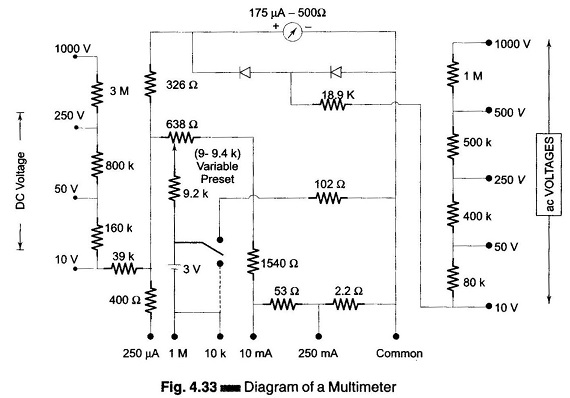Figure 4.33 shows a meter consisting of a dc milliammeter, a dc voltmeter, an ac voltmeter, a microammeter, and an ohmmeter.

### Microammeter:

Figure 4.34 shows a circuit of a multimeter used as a microammeter.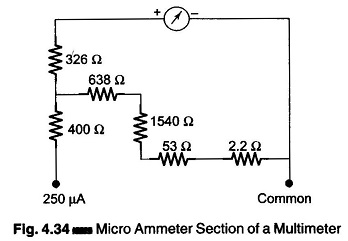### DC Ammeter:

Figure 4.35 shows a working principle of multimeter used as a dc ammeter.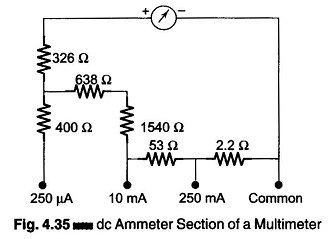### DC Voltmeter:

Figure 4.36 shows the dc voltmeter section of a multimeter.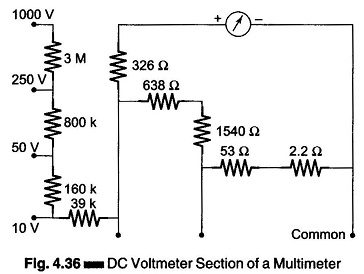### AC Voltmeter:

Figure 4.37 shows the ac voltmeter section of a working principle of multimeter. To measure ac voltage, the output ac voltage is rectified by a half wave rectifier before the current passes through the meter. Across the meter, the other diode serves as protection. The diode conducts when a reverse voltage appears across the diodes, so that current bypasses the meter in the reverse direction.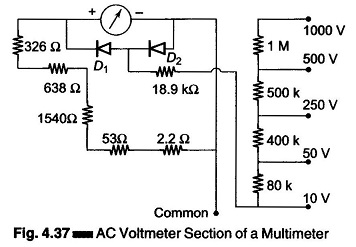### Ohmmeter:

Referring to Fig. 4.38 which shows the ohmmeter section of a multimeter, in the 10 k range the 102 Ω resistance is connected in parallel with the total circuit resistance and in the 1 MΩ range the 102 Ω resistance is totally disconnected from the circuit.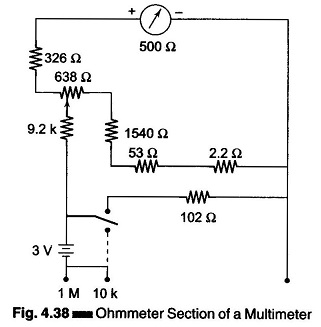Therefore, on the 1 M range the half scale deflection is 10 k. Since on the 10 k range, the 102 Ω resistance is connected across the total resistance, therefore, in this range, the half scale deflection is 100 Ω. The measurement of resistance is done by applying a small voltage installed within the meter. For the 1 M range, the internal resistance is 10 Ω, i.e. value at mid-scale, as shown in Fig. 4.39. And for the 10 k range, the internal resistance is 100 Ω, i.e. value at mid-scale as shown in Fig. 4.40.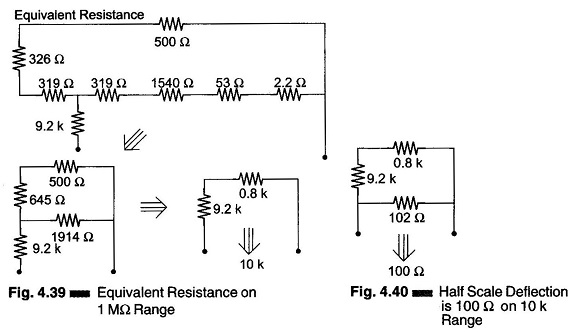The range of an ohmmeter can be changed by connecting the switch to a suitable shunt resistance. By using different values of shunt resistance, different ranges can be obtained.

By increasing the battery voltage and using a suitable shunt, the maximum values which the ohmmeter reads can be changed.

### Multimeter Operating Instructions:

The combination volt-ohm-milliammeter is a basic tool in any electronic laboratory. The proper use of this instrument increases its accuracy and life. The following precautions should be observed.

1. To prevent meter overloading and possible damage when checking voltage or current, start with the highest range of the instrument and move down the range successively.
2. For higher accuracy, the range selected should be such that the deflection falls in the upper half on the meter scale.
3. For maximum accuracy and minimum loading, choose a voltmeter range such that the total voltmeter resistance (ohms per volt x full scale voltage) is at least 100 times the resistance of the circuit under test.
4. Make all resistance readings in the uncrowded portion on the meter scale, whenever possible.
5. Take extra precautions when checking high voltages and checking current in high voltage circuits.
6. Verify the circuit polarity before making a test, particularly when measuring dc current or voltages.
7. When checking resistance in circuits, be sure power to the circuit is switched off, otherwise the voltage across the resistance may damage the meter.
8. Renew ohmmeter batteries frequently to insure accuracy of the resistance scale.
9. Re-calibrate the instrument at frequent intervals.
10. Protect the instrument from dust, moisture, fumes and heat.
Scroll to Top